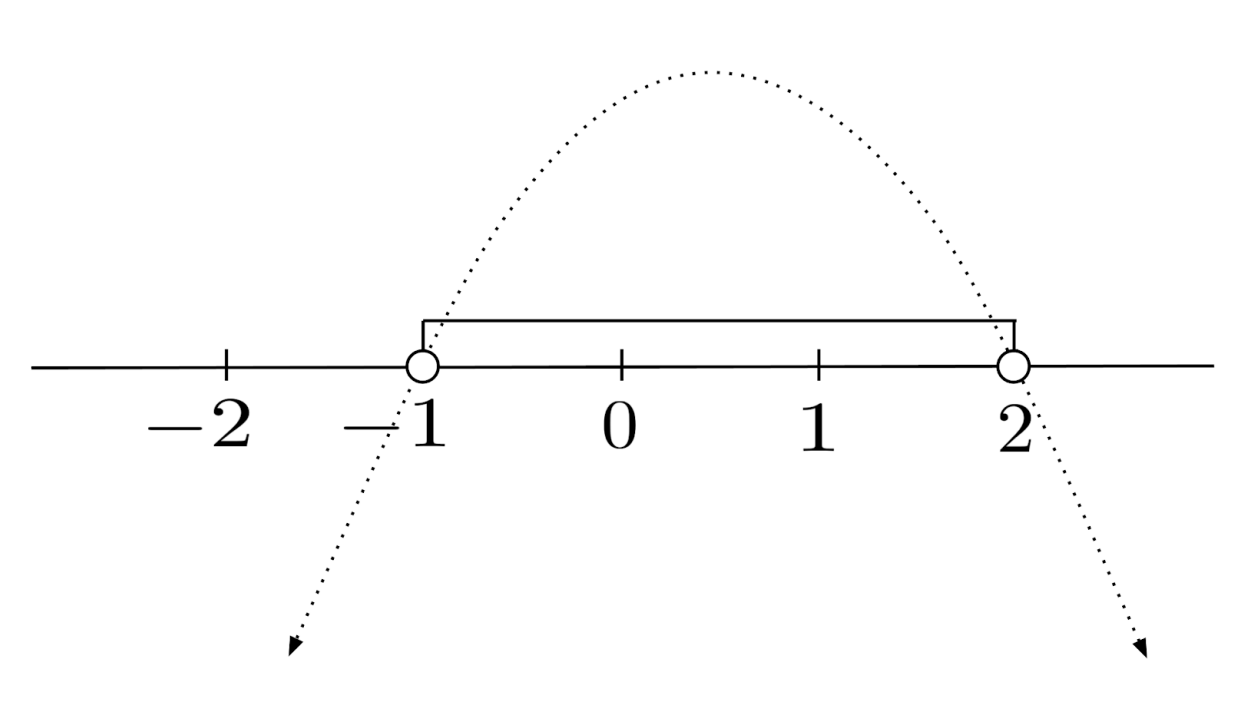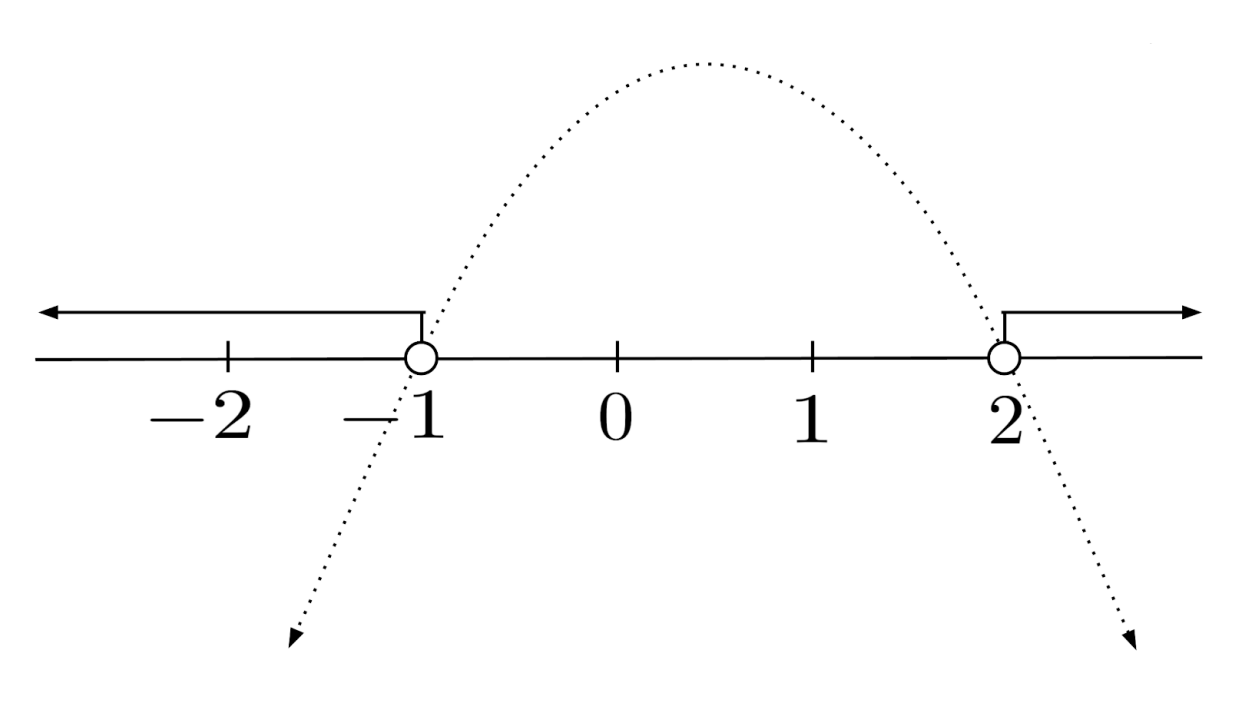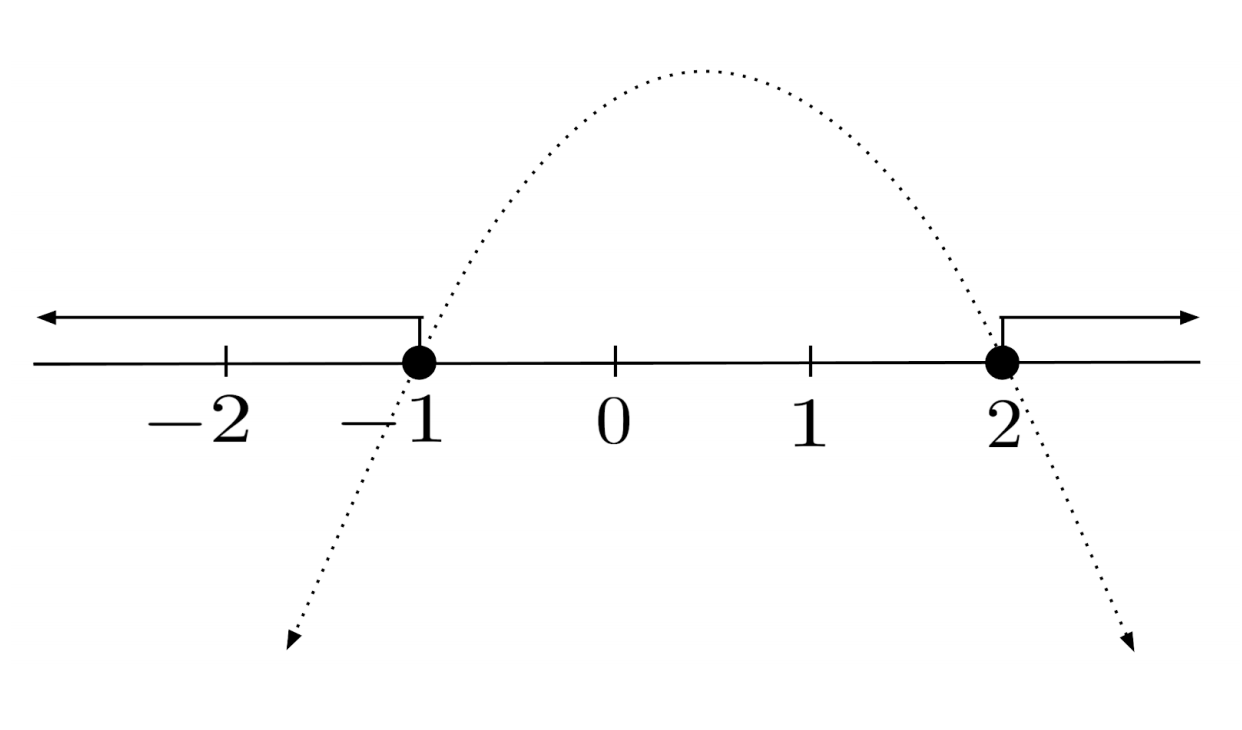Quadratic inequalities are best visualized in the plane.

This post is part of a series.

Quadratic inequalities are best visualized in the plane. For example, to solve a quadratic inequality $-x^2+x+2>0$, we can find the values of $x$ where the parabola $y=-x^2+x+2$ is positive.

Since $y=-x^2+x+2$ is a downward parabola, the solution consists of the values of $x$ in its midsection which arches over the x-axis. That is, the solution consists of all x-values between the solutions to $-x^2+x+2=0$.

This quadratic equation factors to $-(x-2)(x+1)=0$, so the parabola’s midsection is given by $-1<x<2$, or $(-1,2)$ in interval notation.## Case when the Solution is a Union

On the other hand, if we want to solve $-x^2+x+2<0$, then we need to find the values of $x$ where the parabola $y=-x^2+x+2$ is negative.

This time, the solution consists of all the values of $x$ in the arms of the parabola which extend under the x-axis. That is, the solution consists of all x-values less than the leftmost solution or greater than the rightmost solution to $-x^2+x+2=0$.

The solution of the inequality is then given by $x<-1 \text{ or } x>2$, which is $(-\infty, -1) \cup (2, \infty)$ in interval notation. (The $\cup$ symbol is called a union, and it allows us to include multiple segments in interval notation.)To solve $-x^2+x+2 \leq 0$, we just need to propagate the allowance of equality to our final answer. Thus, the solution is $x \leq -1 \text{ or } x \geq 2$, which is $(-\infty, -1] \cup [2,\infty)$ in interval notation.## Case when the Parabola is Never Zero

When a quadratic inequality involves a parabola that is never zero, there is either no solution or the solution is all real numbers.

For example, the parabola $y=x^2+5$ has only positive y-values, so $x^2+5<0$ has no solution and $x^2+5>0$ is solved by all real numbers.

In interval notation, we express all real numbers as the full number line $(-\infty, \infty)$, and we express no solution as $\emptyset$. (The $\emptyset$ symbol is called the empty set, and it represents an interval which doesn’t contain any numbers.)

## Exercises

Solve the following inequalities, writing the solutions in interval notation. (You can view the solution by clicking on the problem.)

$1) \hspace{.5cm} x^2-4 \geq 0$
Solution:
$(-\infty,-2] \cup [2,\infty)$

$2) \hspace{.5cm} x^2+1 > 0$
Solution:
$(-\infty,\infty)$

$3) \hspace{.5cm} -3x^2+27<0$
Solution:
$(-\infty,-3) \cup (3,\infty)$

$4) \hspace{.5cm} x^2-9x+14<0$
Solution:
$(2,7)$

$5) \hspace{.5cm} x^2+x+1 \leq 0$
Solution:
$\emptyset$

$6) \hspace{.5cm} 2x^2 \geq 7x+15$
Solution:
$\left( -\infty, -\frac{3}{2} \right] \cup \left[ 5,\infty \right)$

$7) \hspace{.5cm} x+1 < 6x^2$
Solution:
$\left( -\infty, -\frac{1}{3} \right) \cup \left( \frac{1}{2}, \infty \right)$

$8) \hspace{.5cm} -x^2-7x+30 \geq x^2+7x-30$
Solution:
$(-10,3)$

$9) \hspace{.5cm} 10x(x-3) \leq 7+3x$
Solution:
$\left[ -\frac{1}{5}, \frac{7}{2} \right]$

$10) \hspace{.5cm} (3x+5)^2 \geq 3(x^2-1)+7(x-11)$
Solution:
$\left( -\infty, \infty \right)$

This post is part of a series.

Tags: